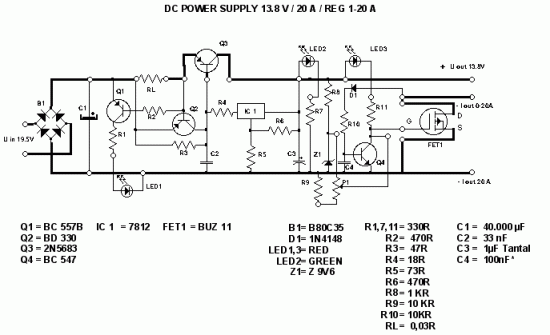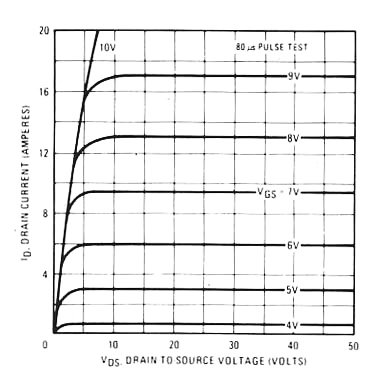## 13 Volt 20 Amp PSU

Description:
This is a Regulated DC power supply with short circuit protection and with current limiter.Notes:
This PSU has been especially designed for current-hungry ham radio transceivers. It delivers safely around 20Amps at 13.8V. For lower currents, a separate current limiting output, capable of 15ma up to a total of 20A has been added.

The power transformer should be capable to deliver at least 25A at 17.5 to 20V. The lower the voltage, the lower power dissipation.

The rectified current will be 'ironed' by the C1, whose capacity should not be less than 40.000uF, (a golden rule of around 2000uF/A), but we recommend 50.000uF. This capacity can be built up by several smaller capacitors in parallel.

The base of this design is a simple 12V regulator (7812). The output voltage can be brought to desired value (here 13.8V) by two external resistors (R5 and R6) using this formula:

U= 12(1+R5/R6)

The low currents (here 15mA) will keep the 7812 in its regular function. As soon as the current rises over 15ma, the voltage drop on R4 will 'open' the Q3, actually handling the high output current. This is a PNP transistor (Ic>25) and current amplification factor of at least 20. The one that has been tested and proven here is the 2N5683.

The current limiting resistance RL, for the maximum output of 20 Amps should be 0.03 Ohms, rated at least 15W. You can use the resistance wire or switch several resistors in parallel, totaling the resistance/power values. Values for other currents can be calculated by the rule:

RL=0.7/Imax

The RL and Q2 (3A PNP such as BD330) form a short circuit 'automatic fuse'. As soon as the maximum current reaches 20Amps, the voltage drop over the resistor RL will open Q2, and thus limit the B-E Current of Q3. Parallel to Q2 is Q1, which lights the LED 1 whenever the current limiting circuit is active. When the 'fuse' is active, the Q2 bridges the R3, so the full current would flow through the IC1, and damage it. Therefore the R4 is inserted, as to limit the IC1 current to 15mA. This makes it possible to run the IC1 without any cooling aid.

The LED 2 will light up every time the PSU is switched on.

There is an adjustable current limiter in parallel to the fixed output, thus providing adjustable current source for smaller currents.

This circuit is very simple too. You will notice that there is no current sensing resistor. But it is really there, in a form of the Rds-on resistance of the N-channel FET, which actually handles the load cutoff from the source. The function of the FET is shown in the diagram 2. When the current Id is rising, the tension Uds over the resistance Rds rises very slowly in the beginning, but very fast after the knick.

This means, that before the knick the FET behaves as a resistor but after it, works as constant current source.

The D2, R3 and B-E connection of the Q4 will sense the Uds voltage of the FET1. When the voltage rises enough, the Q4 will shortcut the FET1 gate to mass, and cut the current flow through the FET 1 off.

However, to enable the FET1 to open, there is certain gate voltage necessary, which in this case is brought up by the voltage divider consisting of R8, Z1, P1 and R9. So the maximum Gate voltage will be the one of the Z1, and the minimal will be around 3V6.

The Z1 voltage (Uz1) will thus determine the max current flowing through the FET 1.

The diagram 2 will show that for 5 Amps the Uz1 should be 5V6, and for 20Amps around 9V6.The Capacitor C4 will determine the 'velocity' or the reaction time of the limiter. 100 uF will make the reaction time to be around 100ms, and 1n will make it 1us.

Within the designed limits, the P1 will limit the current output in the range of 15mA to 20A.

You can use both output simultaneously, but the total output current will be limited by the value of the RL. This PSU can be built also for higher outputs, as long as the transformer will handle the current requirements, and you provide sufficient cooling for the Q3